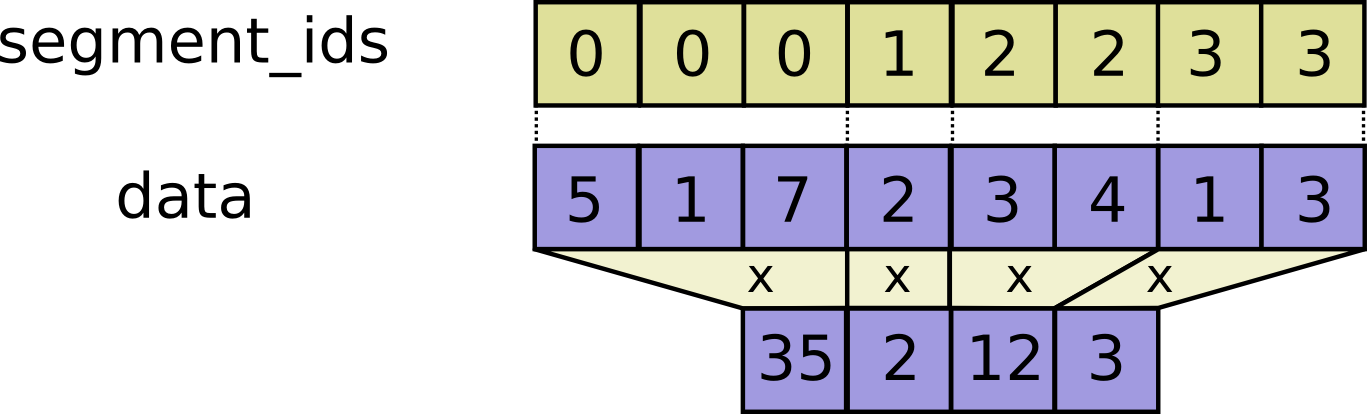# tf.math.segment_prod

Computes the product along segments of a tensor.

tf.math.segment_prod(
data, segment_ids, name=None
)


Read the section on segmentation for an explanation of segments.

Computes a tensor such that $$output_i = \prod_j data_j$$ where the product is over j such that segment_ids[j] == i.

If the product is empty for a given segment ID i, output[i] = 1.#### For example:

c = tf.constant([[1,2,3,4], [4, 3, 2, 1], [5,6,7,8]])
tf.segment_prod(c, tf.constant([0, 0, 1]))
# ==> [[4, 6, 6, 4],
#      [5, 6, 7, 8]]


#### Args:

• data: A Tensor. Must be one of the following types: float32, float64, int32, uint8, int16, int8, complex64, int64, qint8, quint8, qint32, bfloat16, uint16, complex128, half, uint32, uint64.
• segment_ids: A Tensor. Must be one of the following types: int32, int64. A 1-D tensor whose size is equal to the size of data's first dimension. Values should be sorted and can be repeated.
• name: A name for the operation (optional).

#### Returns:

A Tensor. Has the same type as data.